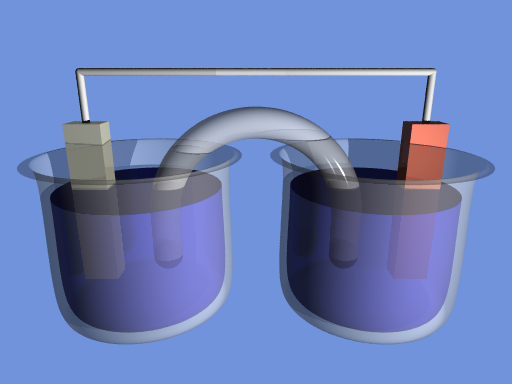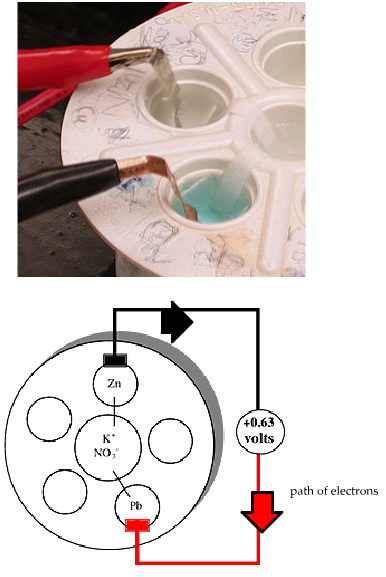# Electrochemistry

-Introduction

Step 1:

-Investigating redox reactions

-Practice with redox reactions

-Reduction tendencies of metal ions

Step 2:

-Electron transfer

-Electrochemical cell

-Practice with cells

-Powering a stopwatch

Step 3:

-Measuring potentials

-Calculating potentials

-Practice with potentials

-Applying potentials

Step 4:

-Non-standard conditions

-Practice with non-standard cells

Feedback Form:

Electrochemistry Tutorial: Galvanic Cells and the Nernst Equation >> Step 2: The Electrochemical Cell

 < Previous | Next >

# Electrochemistry: Galvanic Cells and the Nernst Equation

## Step 2: The Electrochemical Cell

In the movie on the previous page, when a zinc strip is placed in a solution with Cu2+ ions, the following spontaneous redox reaction occurs:

 Zn(s) + Cu2+(aq) --> Zn2+(aq) + Cu(s) Full redox reaction

In this redox reaction, electrons are transferred from Zn to Cu2+. Previously, we wrote half-reactions that illustrate the nature of this electron flow:

 Zn(s) --> Zn2+(aq) + 2 e- Oxidation Cu2+(aq) + 2 e- --> Cu(s) Reduction

The electrochemical cell forces the electrons to flow through a wire as they go from Zn to the Cu2+ ions.

The electrochemical cell consists of two "half-cells" that correspond to each of the above half-cell reactions. For the half-cell corresponding to the oxidation reaction, a strip of Zn metal is placed in a solution of Zn2+ ions. For the reduction half-cell, a strip of Cu metal is placed in a solution of Cu2+ ions. We then connect these cells together (using a wire and a salt bridge) to create an electrical circuit. The following video explains this setup and details the process at a molecular level:

After watching the video, please complete the diagram below to identify the key components in an electrochemical cell:

The following is an electrochemical cell diagram for the reaction shown in the movie above:
Zn(s) + Cu2+(aq) --> Zn2+(aq) + Cu(s).

Match the following labels to their location on the diagram. You will not use all of the labels.Oxidation half-cell
Reduction half-cell
Direction of anion flow in salt bridge >>>
Direction of anion flow in salt bridge <<<
Direction of electron flow >>>
Direction of electron flow <<<
Hint
From the video and the reactions above can you determine which side is the oxidation half-cell and which is the reduction half-cell? The oxidation half-cell is on the left and the reduction half-cell is on the right. Following the reaction, which way do electrons flow through the wire? The electrons flow from the oxidation reaction (Zn(s) --> Zn2+(aq) + 2 e-) to the reduction half-cell (Cu2+(aq) + 2 e- --> Cu(s)) So the electrons flow from the left to the right. Can you identify the flow of ions in the salt bridge? Because negative electrons are flowing through the wire to the reduction half-cell, positive ions travel through the salt bridge to balance the charge of the solution. Which way are negative ions flowing? The labels are (starting from the left and going clockwise): Oxidation half-cell, Direction of electron flow > > >, Direction of anion flow in salt bridge <<< and Reduction half-cell.

Keep in mind that in an electrochemical cell, only the ions travel in solution. The electrons travel between the electrodes on the wire and never enter the solution. The flow of electricity in the circuit is maintained by the electrons moving through the wires and the ions moving through solution.In our laboratory, we will be using a Carrou cell, which provides a more convenient means to carry out experiments on electrochemical cells. A Carrou cell has a number of wells that serve as the above beakers. Each of the outer wells holds a half-cell. The salt bridge connecting two of these outer wells passes through the center well. This is done by filling the center well with a salt solution, and using paper to connect each of the outer wells to the center well. The paper is wet with the salt solution, to ensure ions can flow through the paper. (Please see image to the right).

The images to the right show what a carrou cell looks like in the laboratory, and how we depict it schematically. The schematic shows the cell set up for the spontaneous reaction between Zn metal and Pb2+ ions:

 Zn(s) + Pb2+(aq) --> Zn2+(aq) + Pb(s) Full redox reaction

We can understand the direction of electron flow by breaking this into half-reactions:

 Zn(s) --> Zn2+(aq) + 2 e- Oxidation Pb2+(aq) + 2 e- --> Pb(s) Reduction

Electrons are leaving the Zn half-cell and going towards the Pb half-cell. A convenient short-hand notation for this is:

Zn(s)| Zn2+(aq) || Pb2+(aq) | Pb(s)

In this notation, the anode, or oxidation half-cell is on the left and the cathode, or reduction half-cell is on the right. The double vertical line, ||, represents the salt bridge, and the single vertical line represents the phase boundary between a solid metal and its salt solution.

A voltmeter is used to measure the potential, or voltage, of an electrochemical cell. Voltmeters have a positive and negative terminal and, by convention, a red wire is connected to positive terminal and a black wire is connected to the negative terminal. The sign of the reading on the voltmeter tells us the spontaneous direction of the electron flow. In the cell: Zn(s)| Zn2+(aq) || Pb2+(aq) | Pb(s), the spontaneous redox reaction corresponds to flow of electrons from Zn to the Pb half-cell. If the black wire is connected to the Zn electrode and the red wire is connected to the Pb electrode, then the voltmeter will show a positive value. If we reverse this, by connecting the black wire to the Pb electrode and the red wire to the Zn electrode, then the voltmeter will show a negative value. Remember: if the voltmeter is showing a positive value, then the spontaneous direction of electron flow is from the black wire to the red wire. A commonly used language in electrochemistry is that of anode and cathode. Electrons flow from the anode (electron provider or electron source) to the cathode (electron receiver or electron sink). If the voltmeter has a positive reading, the black wire is on the anode and the red wire is on the cathode.

 < Previous | Next >